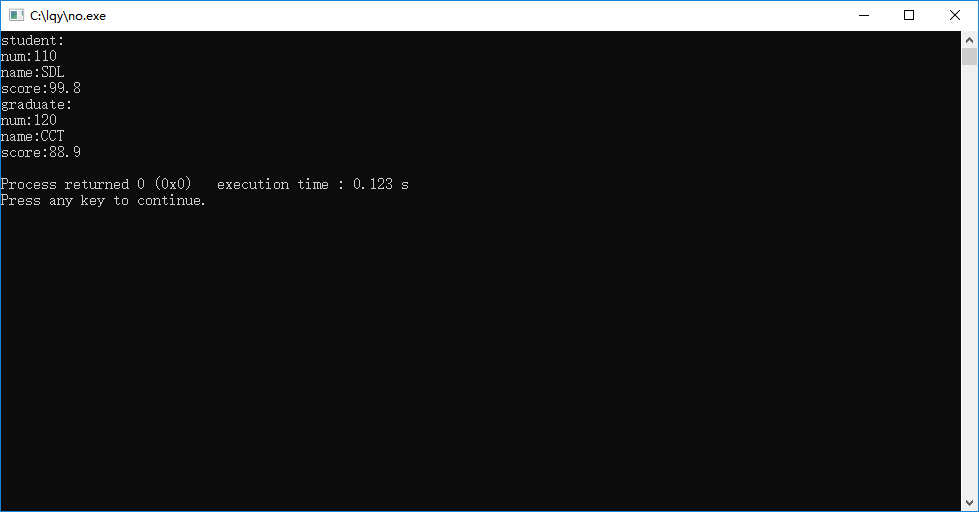# C++

---恢复内容开始---

重载与多态

c++中预定义的运算符的操作对象只能是基本数据类型。实际上，对于很多用户自定义类型（比如类），同样也需要类似的操作。

我在这里举一个非常经典例子，复数运算。

class Complex
{
public:
Complex(double r=0.0,double i=0.0):real(r),imag(i){}
void display() const;
private:
double real;
double imag;
};

运算符与类结合，产生新的含义。

作用：为了实现类的多态性(多态是指一个函数名有多种含义)

1、运算符的操作需要修改类对象的状态，则使用成员函数。如需要做左值操作数的运算符（如=，+=，++）

2、运算时，有数和对象的混合运算时，必须使用友元

3、二元运算符中，第一个操作数为非对象时，必须使用友元函数。如输入输出运算符<<和>>

 运算符 建议使用 所有一元运算符 成员函数 ＝ ( ) [ ]  -> 必须是成员函数 += -= /= *= ^= &= != %= >>= <<= , 似乎带等号的都在这里了 成员函数 所有其它二元运算符, 例如: –,+,*,/ 友元函数 << >> 必须是友元函数

2. 参数和返回值

当参数不会被改变，一般按const引用来传递(若是使用成员函数重载，函数也为const).

对于返回数值的决定:

1) 如果返回值可能出现在=号左边, 则只能作为左值, 返回非const引用。

2) 如果返回值只能出现在=号右边, 则只需作为右值, 返回const型引用或者const型值。

3) 如果返回值既可能出现在=号左边或者右边, 则其返回值须作为左值, 返回非const引用。

+和 -运算符的重载:

class Point
{
private:
int x;
public:
Point(int x1)
{      x=x1;}
Point(Point& p)
{      x=p.x;}
const Point operator+(const Point& p);//使用成员函数重载加号运算符
friend const Point operator-(const Point& p1,const Point& p2);//使用友元函数重载减号运算符
};

const Point Point::operator+(const Point& p)
{
return Point(x+p.x);
}

Point const operator-(const Point& p1,const Point& p2)
{
return Point(p1.x-p2.x);
}

1.使用虚函数实现运行时的多态性的关键在于：必须通过基类指针访问这些函数。

2. 一旦一个函数定义为虚函数，无论它传下去多少层，一直保持为虚函数。

l纯虚函数定义的一般形式：

Virtual type func_name(参数列表)=0;

l  含有纯虚函数的基类称为抽象基类。抽象基类又一个重要特性：抽象类不能建立对象。但是抽象基类可以有指向自己的指针，以支持运行时的多态性。

#include<iostream>
#include<string>

using namespace std;

class Student
{
public:
Student(int , string, float);
void display();
protected:
int num;
string name;
float score;
};
Student::Student(int n, string na, float s)
{
num=n;
name=na;
score=s;
}
void Student::display()
{
cout<<"num:"<<num<<"\nname:"<<name<<"\nscore:"<<score<<endl;
}
{
public:
Graduate(int , string , float, float);
void display();

private:
float pay;

};
{
}
{
cout<<"num:"<<num<<"\nname:"<<name<<"\nscore:"<<score<<"\npay:"<<pay<<endl;
}

int main()
{
Student stud1(110,"SDL",99.8);

Student *p;

p=&stud1;
cout<<"student:"<<endl;
p->display();
p->display();

return 0;
}-恢复内容结束---

---恢复内容结束---

posted @ 2019-10-27 19:01  ****l****  阅读(660)  评论(0编辑  收藏  举报